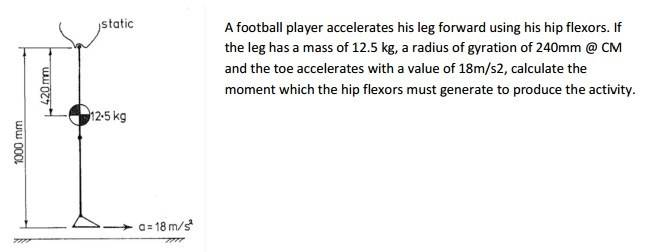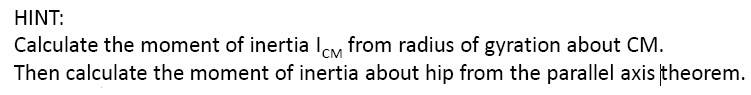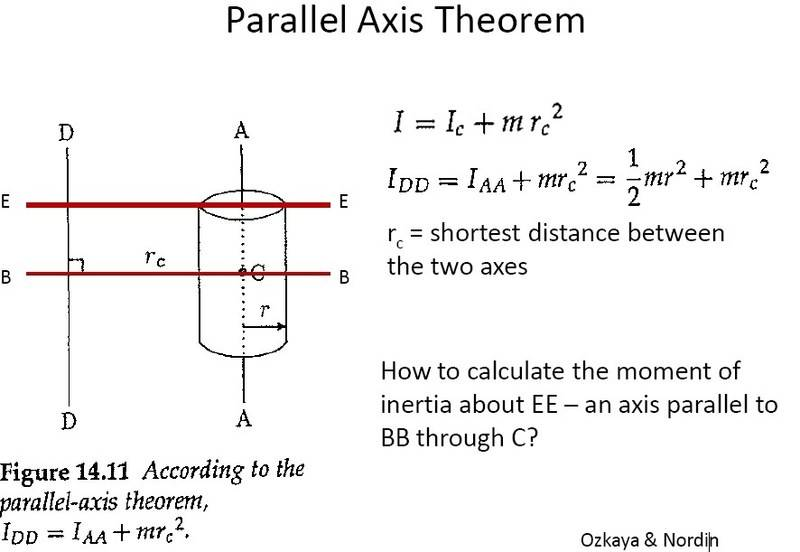# Calculating a moment required, using radius of gyration and parallel axis theorem

## Homework Statement## Homework Equations

r = root (I/m)
I = moment of inertia
m = mass

parallel axis theorem given above

## The Attempt at a Solution

Okay, so I think the moment about CM is just m*0.24^2, but after that, I'm less sure.
Is the moment about the hip just m*0.42^2 + m*0.24^2?

If so, it's taking the acceleration and the distance to the toe into account that I'm having difficulty with. Is it just F = ma? If so, is the m taken from the moment of inertia at the hip, or the toe? I figure the distance to the toe must be significant, but I don't know how to account for it.

Any help?

Doc Al
Mentor
Okay, so I think the moment about CM is just m*0.24^2, but after that, I'm less sure.
Is the moment about the hip just m*0.42^2 + m*0.24^2?
Yes, you've got it.

If so, it's taking the acceleration and the distance to the toe into account that I'm having difficulty with. Is it just F = ma? If so, is the m taken from the moment of inertia at the hip, or the toe?
How would you write the equivalent of F = ma for rotational motion?
I figure the distance to the toe must be significant, but I don't know how to account for it.
Given the tangential acceleration, how would you find the angular acceleration?

Yes, you've got it.

How would you write the equivalent of F = ma for rotational motion?

Given the tangential acceleration, how would you find the angular acceleration?

Okay, maybe it's best to do the last two bits in reverse order;
if the tangential acceleration is 18m/s^2, and the radius is 1m, is the angular acceleration also 18 radians/s^2?

Then is the moment: angular acceleration * the moment about the hip?

Doc Al
Mentor
Okay, maybe it's best to do the last two bits in reverse order;
if the tangential acceleration is 18m/s^2, and the radius is 1m, is the angular acceleration also 18 radians/s^2?
Right!

Then is the moment: angular acceleration * the moment about the hip?